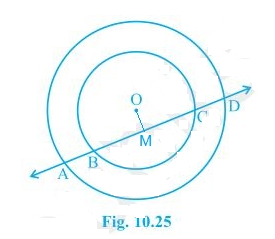Guru

# If a line intersects two concentric circles (circles with the same centre) with centre O at A, B, C and D, prove that AB = CD (see Fig. 10.25). Q.4

• 0

Please give me the best way for solving the problem of class 9th ncert math of Circles chapter of math of class 9th of exercise 10.4 of question no 4  what is the tricky way for solving this question If a line intersects two concentric circles (circles with the same centre) with centre O at A, B, C and D, prove that AB = CD (see Fig. 10.25).

Share

1. The given image is as follows:First, draw a line segment from O to AD such that OM ⊥ AD.

So, now OM is bisecting AD since OM ⊥ AD.

Therefore, AM = MD — (i)

Also, since OM ⊥ BC, OM bisects BC.

Therefore, BM = MC — (ii)

From equation (i) and equation (ii),

AM-BM = MD-MC

∴ AB = CD

• 0